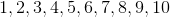# Common Core: Kindergarten Math : Counting & Cardinality

## Example Questions

1 2 50 51 52 53 54 55 56 58 Next →

### Example Question #115 : Comparing Two Numbers Between 1 And 10: Ccss.Math.Content.K.Cc.C.7

Fill in the blank.__________is greater than

is equal to

is less than

is greater than

Explanation:is greater thanbecausecomes afterwhen we are counting, which means it is greater.### Example Question #116 : Comparing Two Numbers Between 1 And 10: Ccss.Math.Content.K.Cc.C.7

Fill in the blank.__________is greater than

is equal to

is less than

is greater than

Explanation:is greater thanbecausecomes afterwhen we are counting, which means it is greater.### Example Question #117 : Comparing Two Numbers Between 1 And 10: Ccss.Math.Content.K.Cc.C.7

Fill in the blank.__________is less than

is greater than

is equal to

is greater than

Explanation:is greater thanbecausecomes afterwhen we are counting, which means it is greater.### Example Question #118 : Comparing Two Numbers Between 1 And 10: Ccss.Math.Content.K.Cc.C.7

Fill in the blank.__________is equal to

is less than

is greater than

is greater than

Explanation:is greater thanbecausecomes afterwhen we are counting, which means it is greater.1 2 50 51 52 53 54 55 56 58 Next →

### All Common Core: Kindergarten Math Resources